x=j (x)

f1(x)=f2(x)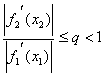首先从一个近似根x0出发（x0可由图解法粗略估计出），代入方程右边，解方程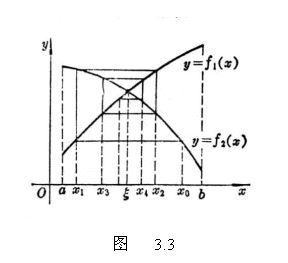f1(x)=f2(x0)

f1(x)=f2(x1)

f1(x)=f2(xn)

x0,  x1,  x2,L, xn,L

收敛速度（即误差消失速度）与an相当，而

a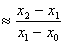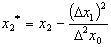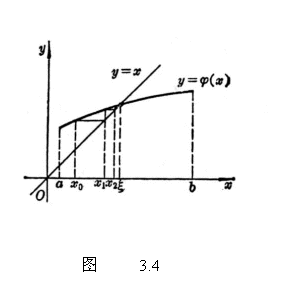对于方程x=j (x)，只要j(x)[a,b]上连续，且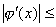q<1，那末，它的根可由

x1=j (x0)

x2=j (x1)

LLLL

xn+1=j (xn)